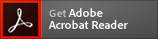Colistin sodium methanesulfonate A cyclopolypeptide antibiotic and derivative of Polymyxin E

# Colistin sodium methanesulfonate (CAS 8068-28-8)

Colistin sodium methanesulfonate | CAS 8068-28-8 is rated 5.0 out of 5 by 1.
Synonym: Colimycin sodium methanesulfonate; Methanesulfonic acid derivative of Polymyxin E; Polymyxin E sodium methanesulfonate
Application: A cyclopolypeptide antibiotic and derivative of Polymyxin E
CAS Number: 8068-28-8
Molecular Weight: 1759.90
Molecular Formula: C58H115N16O28S55Na
* Refer to Certificate of Analysis for lot specific data (including water content).Binds to lipids on the cell cytoplasmic membrane of Gram-negative bacteria and disrupts the cell wall integrity. Antimicrobial spectrum: Gram-negative bacteria. Cyclopolypeptide antibiotic. Endotoxin-binding capacity.

#### References

1. M.J. Rogers, J. Cohen Infection (Munich) 14, 79, (1986)

Physical State :
Solid
Solubility :
Soluble in water (10 mg/ml).
Storage :
Store at 4° C
For Research Use Only. Not Intended for Diagnostic or Therapeutic Use.
WGK Germany :
2
RTECS :
GH1650000
PubChem CID :
16211791
Merck Index :
14: 2479
MDL Number :
MFCD00130824
EC Number :
232-516-9
SMILES :
CCC(C)CCCCC(=O)N[C@@H](CCN)C(=O)N[C@@H]([C@@H](C)O)C(=O)N[C@@H](CCN)C(=O)N[C@H]1CCNC(=O)[C@@H](NC(=O)[C@@H](NC(=O)[C@H](NC(=O)[C@@H](NC(=O)[C@H](NC(=O)[C@@H](NC1=O)CCN)CC(C)C)CC(C)C)CCN)CCN)[C@@H](C)O.CS(=O)(=O)[O-].CS(=O)(=O)[O-].CS(=O)(=O)[O-].CS(=O)(=O)[O-].CS(=O)(=O)[O-].[Na+].[Na+].[Na+].[Na+].[Na+]